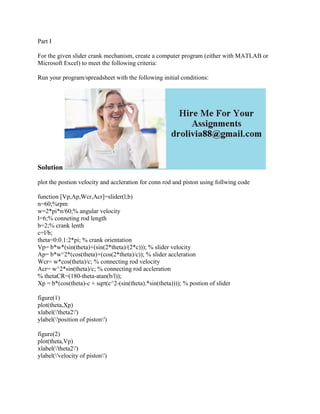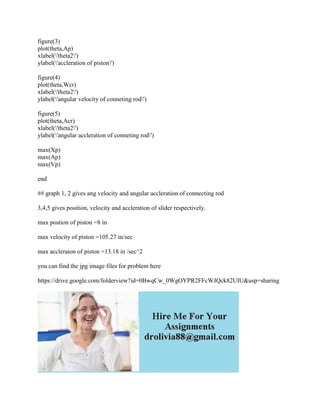Seu SlideShare está sendo baixado. ×

# Part I For the given slider crank mechanism- create a computer program (1).docx

Anúncio
Anúncio
Anúncio
Anúncio
Anúncio
Anúncio
Anúncio
Anúncio
Anúncio
Anúncio
AnúncioCarregando em…3
×

1 de 2 Anúncio

# Part I For the given slider crank mechanism- create a computer program (1).docx

Part I
For the given slider crank mechanism, create a computer program (either with MATLAB or Microsoft Excel) to meet the following criteria:
Solution
plot the postion velocity and accleration for conn rod and piston using follwing code
function [Vp,Ap,Wcr,Acr]=slider(l,b)
n=60;%rpm
w=2*pi*n/60;% angular velocity
l=6;% conneting rod length
b=2;% crank lenth
c=l/b;
theta=0:0.1:2*pi; % crank orientation
Vp= b*w*(sin(theta)+(sin(2*theta)/(2*c))); % slider velocity
Ap= b*w^2*(cos(theta)+(cos(2*theta)/c)); % slider accleration
Wcr= w*cos(theta)/c; % connecting rod velocity
Acr= w^2*sin(theta)/c; % connecting rod accleration
% thetaCR=(180-theta-atan(b/l));
Xp = b*(cos(theta)-c + sqrt(c^2-(sin(theta).*sin(theta)))); % postion of slider
figure(1)
plot(theta,Xp)
xlabel(\'theta2\')
ylabel(\'position of piston\')
figure(2)
plot(theta,Vp)
xlabel(\'theta2\')
ylabel(\'velocity of piston\')
figure(3)
plot(theta,Ap)
xlabel(\'theta2\')
ylabel(\'accleration of piston\')
figure(4)
plot(theta,Wcr)
xlabel(\'theta2\')
ylabel(\'angular velocity of conneting rod\')
figure(5)
plot(theta,Acr)
xlabel(\'theta2\')
ylabel(\'angular accleration of conneting rod\')
max(Xp)
max(Ap)
max(Vp)
end
## graph 1, 2 gives ang velocity and angular accleration of connecting rod
3,4,5 gives position, velocity and accleration of slider respectively.
max postion of piston =8 in
max velocity of piston =105.27 in/sec
max accleraion of piston =13.18 in /sec^2
you can find the jpg image files for problem here
.

Part I
For the given slider crank mechanism, create a computer program (either with MATLAB or Microsoft Excel) to meet the following criteria:
Solution
plot the postion velocity and accleration for conn rod and piston using follwing code
function [Vp,Ap,Wcr,Acr]=slider(l,b)
n=60;%rpm
w=2*pi*n/60;% angular velocity
l=6;% conneting rod length
b=2;% crank lenth
c=l/b;
theta=0:0.1:2*pi; % crank orientation
Vp= b*w*(sin(theta)+(sin(2*theta)/(2*c))); % slider velocity
Ap= b*w^2*(cos(theta)+(cos(2*theta)/c)); % slider accleration
Wcr= w*cos(theta)/c; % connecting rod velocity
Acr= w^2*sin(theta)/c; % connecting rod accleration
% thetaCR=(180-theta-atan(b/l));
Xp = b*(cos(theta)-c + sqrt(c^2-(sin(theta).*sin(theta)))); % postion of slider
figure(1)
plot(theta,Xp)
xlabel(\'theta2\')
ylabel(\'position of piston\')
figure(2)
plot(theta,Vp)
xlabel(\'theta2\')
ylabel(\'velocity of piston\')
figure(3)
plot(theta,Ap)
xlabel(\'theta2\')
ylabel(\'accleration of piston\')
figure(4)
plot(theta,Wcr)
xlabel(\'theta2\')
ylabel(\'angular velocity of conneting rod\')
figure(5)
plot(theta,Acr)
xlabel(\'theta2\')
ylabel(\'angular accleration of conneting rod\')
max(Xp)
max(Ap)
max(Vp)
end
## graph 1, 2 gives ang velocity and angular accleration of connecting rod
3,4,5 gives position, velocity and accleration of slider respectively.
max postion of piston =8 in
max velocity of piston =105.27 in/sec
max accleraion of piston =13.18 in /sec^2
you can find the jpg image files for problem here